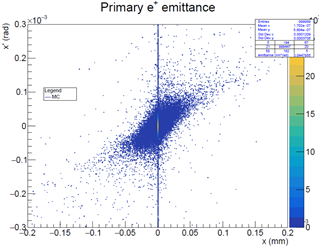# Get primary particle production angle

Hello, here my beam

``````/gps/particle e+
/gps/energy 45 GeV
/gps/ene/type Gauss
/gps/ene/sigma 0 GeV
/gps/pos/type Beam
/gps/pos/shape Circle
/gps/pos/sigma_x 0.3 mm
/gps/pos/sigma_y 0.3 mm
/gps/pos/centre 0 0 -100 cm
/gps/direction 0 0 1
``````

to get the vertex of a primary particle outgoing my target I do

``````if(NextVol && ThisVol->GetName()=="Envelope" && NextVol->GetName()=="World" && step->GetTrack()->GetParentID()==00 && step->GetTrack()->GetDynamicParticle()->GetPDGcode()==-11) {
...

`step->GetTrack()->GetVertexPosition().x();`
``````

(and similiarly for y and z)
Is it possible to get the vertex releated to the angles of primary particles? I mean…the beam should be just along z…but I want to check it …so I want to calculate the angles with x and y axes of primary particles. is it possible?

I’m asking it because when I plot the angle of primary particles (x’=atan px/pz) outgoing the target in function of
x=x_ext- xprod (where x_ext is the x position at the exit of the target and x_prod is the x position of e^+ production ) I get this plotso I don’t know if maybe positrons have an angle with the x axis

1 Like